# 神经网络原来这么简单，机器学习入门贴送给你 | 干货

“神经网络”一词很流行，人们通常认为它很难，但其实要简单得多。

1、每个输入乘以相应的权重；2、将所有加权输入加在一起，在加上一个偏差b；3、导入一个激活函数，得到输出y。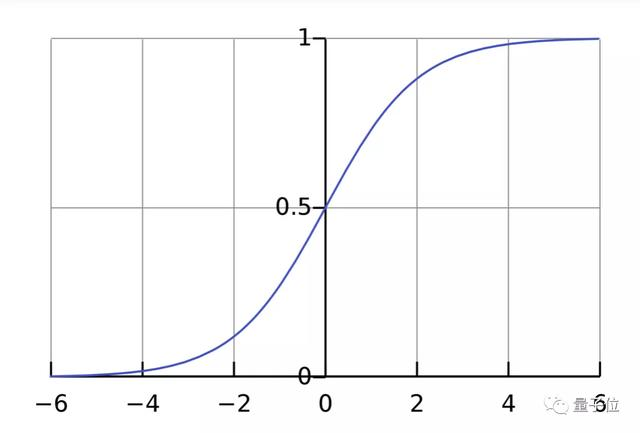input：x=[2,3]

output：y=0.999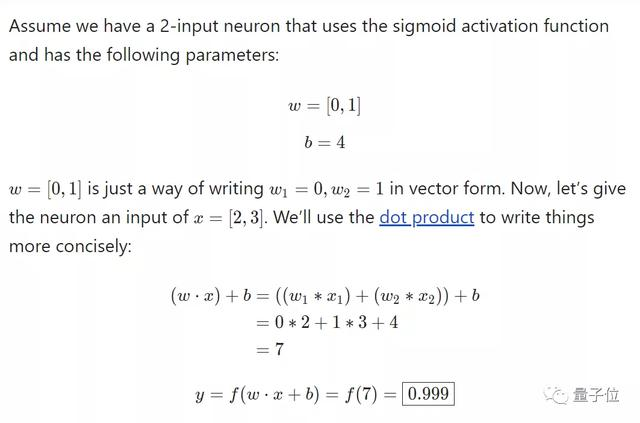import numpy as np  def sigmoid(x):   # Our activation function: f(x) = 1 / (1 + e^(-x))   return 1 / (1 + np.exp(-x))  class Neuron:   def __init__(self, weights, bias):     self.weights = weights     self.bias = bias    def feedforward(self, inputs):     # Weight inputs, add bias, then use the activation function     total = np.dot(self.weights, inputs) + self.bias     return sigmoid(total)  weights = np.array([0, 1]) # w1 = 0, w2 = 1 bias = 4                   # b = 4 n = Neuron(weights, bias)  x = np.array([2, 3])       # x1 = 2, x2 = 3 print(n.feedforward(x))    # 0.9990889488055994   构建神经网络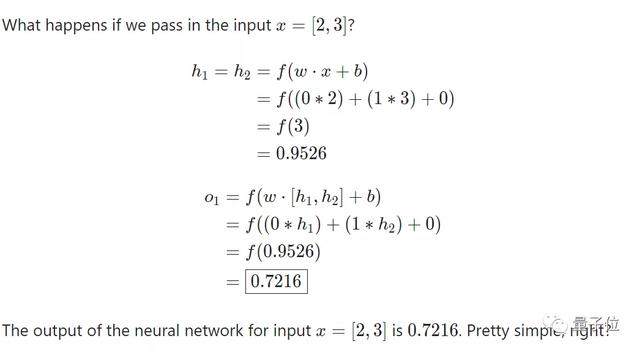import numpy as np  # ... code from previous section here  class OurNeuralNetwork:   '''   A neural network with:     - 2 inputs     - a hidden layer with 2 neurons (h1, h2)     - an output layer with 1 neuron (o1)   Each neuron has the same weights and bias:     - w = [0, 1]     - b = 0   '''   def __init__(self):     weights = np.array([0, 1])     bias = 0      # The Neuron class here is from the previous section     self.h1 = Neuron(weights, bias)     self.h2 = Neuron(weights, bias)     self.o1 = Neuron(weights, bias)    def feedforward(self, x):     out_h1 = self.h1.feedforward(x)     out_h2 = self.h2.feedforward(x)      # The inputs for o1 are the outputs from h1 and h2     out_o1 = self.o1.feedforward(np.array([out_h1, out_h2]))      return out_o1  network = OurNeuralNetwork() x = np.array([2, 3]) print(network.feedforward(x)) # 0.7216325609518421  训练神经网路——计算损失函数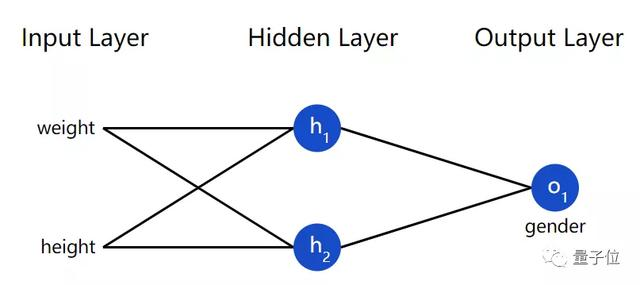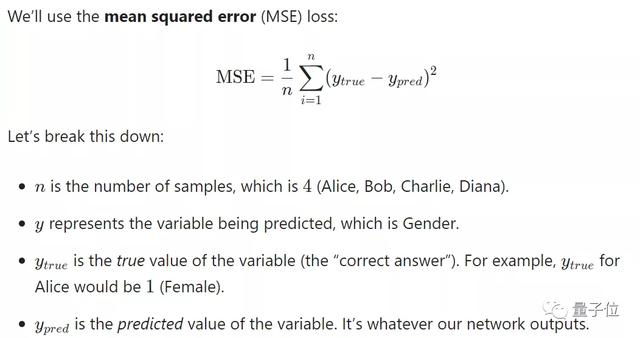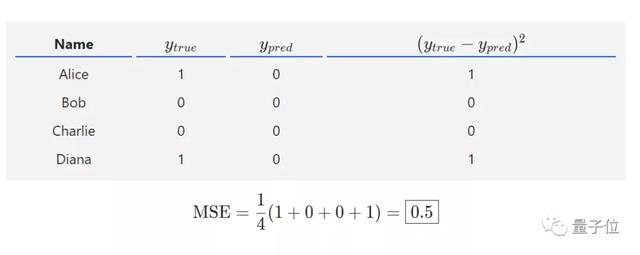Python示例：

import numpy as np  def mse_loss(y_true, y_pred):   # y_true and y_pred are numpy arrays of the same length.   return ((y_true - y_pred) ** 2).mean()  y_true = np.array([1, 0, 0, 1]) y_pred = np.array([0, 0, 0, 0])  print(mse_loss(y_true, y_pred)) # 0.5  训练神经网络——最小化损失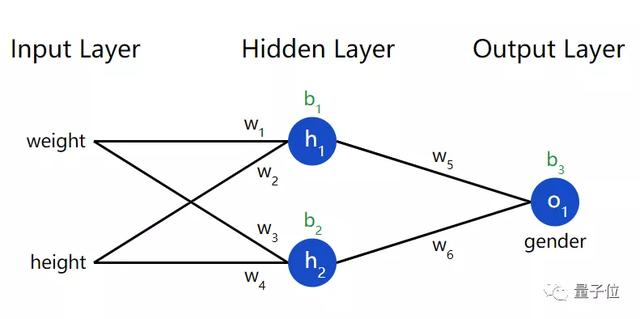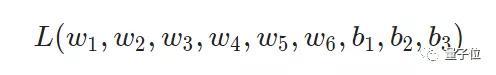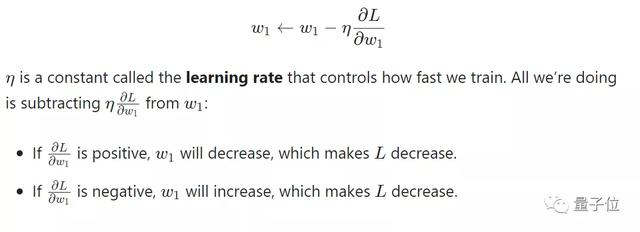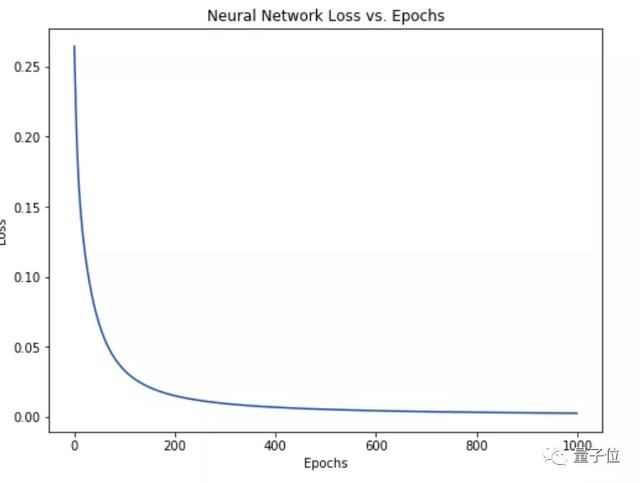1、数据集中选择一个样本，就如Alice。

2、利用反向传播计算所有变量的偏导数。

3、使用随机梯度下降来训练神经网络，更新变量。

4、返回步骤1。

## 官方微博/微信↑扫描二维码

1.用手机扫左侧二维码；

2.在添加朋友里，搜索关注TechWeb。